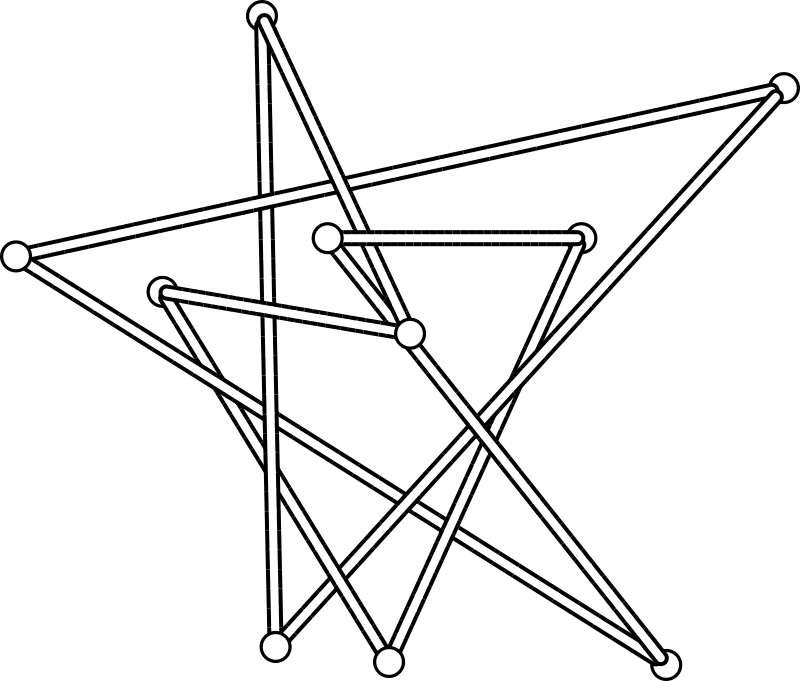# Clayton ShonkwilerThe paper “New stick number bounds from random sampling of confined polygons”, written with Thomas D. Eddy, finds new upper bounds on the stick number of more than 40% of the 219 knots up to 10 crossings for which is was previously unknown.

The stick number of a knot is the minimum number of straight segments needed to construct a polygonal version of the knot. This is a simple geometric knot invariant which is extremely hard to compute: in most cases the best we can do is find an upper bound for the stick number by constructing examples.

In the paper, we use the symplectic approach to sampling equilateral random polygons in confinement introduced in joint work with Jason Cantarella to generate very large ensembles of polygons and then determined the knot type of each polygon. We ended up generating a total of 220 billion random polygons for this work.

The above image shows an equilateral 10-stick $$10_{16}$$ knot. This beats Rawdon and Scharein’s record by 2: they could only find a 12-stick equilateral $$10_{16}$$.